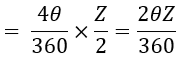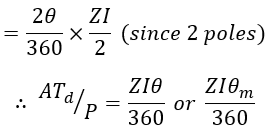# Demagnetising and Cross-magnetising Conductors & its Equation

When the field winding of a dc generator is excited, a flux with uniform distribution in the air gap of the dc generator is produced. Once the generator is loaded, load current flows through the armature conductors and set up its own flux. This armature flux will be always in the 180° phase apart from the field flux and distorts or opposes the uniformly distributed main field flux.

The opposition of armature flux on the main field flux is called armature reaction. This armature reaction has two undesirable effects, either it weakens (reduces) the main field flux called 'Demagnetising Effect' or it distorts the main field flux called 'Cross-magnetising Effect'.

## Phenomenon of Demagnetisation and Cross-magnetisation :

Assume, for example, the brushes are lying at the new MNA position (to avoid sparking during commutation). Let θ be the angle between GNA and MNA. The armature MMF will now lie along with the new position of the brush axis as shown in the below diagram.

Suppose the field winding flux is in the direction from N to S i.e., top to bottom. We can see that the current carrying armature conductors in the region AOC and BOD are in the opposite direction to the field winding flux.

Thus armature field Fa by the conductors in the region AOC (θ + θ = 2θ) and BOD (θ + θ = 2θ) exerts demagnetising influence on the main field Ff (reduces field strength) and called demagnetising armature conductors or weakening component of the armature reaction.

Similarly, the remaining armature conductors i.e., in the region AOD and BOC, produce a flux Fa in such a direction that right angle to the field flux Ff as shown above. Thus component OFa is at right angles to the vector OFf as shown in the vector representation.

These armature conductors (in the region AOD and BOC) produce a distorted effect on flux distribution in the air gap produced by the main field. This distortion effect by the armature conductors is known as cross-magnetising armature conductors or a distorting component of the armature reaction.

## Equation for Demagnetisation and Cross-magnetisation Conductors :

#### Let,ATd = Demagnetising ampere-turns.ATc = Cross-magnetising ampere-turns.I = Current in each armature conductor (Ia/2 for wave winding or Ia/P for lap winding).Z = Total number of armature conductors.θ = Forward mechanical lead of brush (degrees).

The conductors responsible for the demagnetising effect are lying in the region AOC and BOD i.e. 2θ + 2θ = 4θ. The total number of armature conductor in angle AOC and BOD (i.e. 4θ) is given by,

#### Total turns in 4θ (since 1 turn = 2 conductors),Demagnetising ampere-turns in 4θ,Demagnetising ampere-turns per pole,The demagnetising effect in a dc generator can be neutralized by adding a number of extra ampere-turns. The extra ampere-turns add per-pole is given as,

The armature conductors responsible for cross-magnetising effect are lying in the region AOD and BOC. We know that the total ampere-turns per pole = ZI/2P (i.e, combination of demagnetising & cross-magnetising ampere-turns). Now by subtracting the equation of demagnetising amper-turns per-pole from total ampere-turns per-pole gives the equation for cross-magnetising ampere-turns per-pole.

i.e., Cross-magnetising ampere turns/pole = Total ampere-turns/pole - ATd/pole

While determining the value of demagnetising and cross-magnetising ampere-turns the angle θ is considered in mechanical degrees. The below expression shows the relation between electrical and mechanical degrees.

Do not enter any spam links and messages